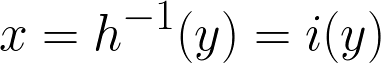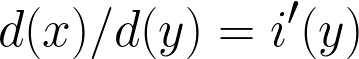Let X be random variable with  a probability density function pdfx=f(x)  and cumulative density function of cdfx=F(x)

b=f(a)

Let Y be random variable with a  probability density function pdfy=g(y) for every y.

Let Y=h(X)  and  h is monotone.

Let y=h(x)we know that F(x)=However, the total chance of something less than y to happen is equal to the total chance of something less than x to happen.

This is because whatever percentage of time X end up to be less than, Y endup to be less than y.

then cdfy G(y) must be equal to F(x)\frac{d G(y)}{d(y)}=f(x) \frac {dx}{dy}

orG(y)=\int_{-\infty }^{y}f(i(y)) {i}'(y) dy\frac{d G(y)}{dy}=f(x) \frac {i'{(y)}dy}{dy}=f(x) i'{(y)}

This meansg(y)=f(x) \frac {dx}{dy}=f(x) i'{(y)}

Freund, J. E. (1962). Mathematical statistics. Englewood Cliffs, N.J.: Prentice-Hall.   (page 133)